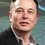This problem

I recently learnt a way to tackle these types of problems - Here I Learnt it, explained to me by @Pranjal Jain

$f(x) = x^4 + ax^3 + bx^2 + cx + d$

We can observe that -

$f(e) = e\times1993$

thus - $f(11) - 11\times1993 = 0$ , $f(-7) + 7\times1993 = 0$

$f(11) + f(-7) = 1993(11 - 7) = 1993\times4$

$\dfrac{f(11) + f(-7)}{4} = 1993$

Where I am wrong , please don't provide me the answer , just comment where I am wrong.

Did I started wrongly i.e - $f(e) = e\times1993$ ?

Thank youNote by U Z
6 years, 7 months ago

This discussion board is a place to discuss our Daily Challenges and the math and science related to those challenges. Explanations are more than just a solution — they should explain the steps and thinking strategies that you used to obtain the solution. Comments should further the discussion of math and science.

When posting on Brilliant:

• Use the emojis to react to an explanation, whether you're congratulating a job well done , or just really confused .
• Ask specific questions about the challenge or the steps in somebody's explanation. Well-posed questions can add a lot to the discussion, but posting "I don't understand!" doesn't help anyone.
• Try to contribute something new to the discussion, whether it is an extension, generalization or other idea related to the challenge.

MarkdownAppears as
*italics* or _italics_ italics
**bold** or __bold__ bold
- bulleted- list
• bulleted
• list
1. numbered2. list
1. numbered
2. list
Note: you must add a full line of space before and after lists for them to show up correctly
paragraph 1paragraph 2

paragraph 1

paragraph 2

[example link](https://brilliant.org)example link
> This is a quote
This is a quote
    # I indented these lines
# 4 spaces, and now they show
# up as a code block.

print "hello world"
# I indented these lines
# 4 spaces, and now they show
# up as a code block.

print "hello world"
MathAppears as
Remember to wrap math in $$ ... $$ or $ ... $ to ensure proper formatting.
2 \times 3 $2 \times 3$
2^{34} $2^{34}$
a_{i-1} $a_{i-1}$
\frac{2}{3} $\frac{2}{3}$
\sqrt{2} $\sqrt{2}$
\sum_{i=1}^3 $\sum_{i=1}^3$
\sin \theta $\sin \theta$
\boxed{123} $\boxed{123}$

Sort by:

$f(x) \neq 1993x, x \in \mathbb R$

- 6 years, 7 months ago

What is your reason behind the claim f(e)=1993e

- 6 years, 7 months ago

Sorry I misunderstood it

- 6 years, 7 months ago

Wanna know the Wolfram|Alpha approach for this?

- 6 years, 7 months ago

nope

- 6 years, 7 months ago

@U Z Hmm, I wish more people had read A=B and were exposed to EM

- 6 years, 7 months ago

Should I delete this note because in a way I am discussing a problem

- 6 years, 7 months ago

@U Z Just delete that solution comment

- 6 years, 7 months ago

Thank you! because of your note I also got a chance to learn .

- 6 years, 7 months ago

Hey! You misunderstood it!! See. $f(x)-1993x$ has roots $1, 2$ and $3$. So we can say that $f(x)-1993x=(x-1)(x-2)(x-3)(x-\alpha)$ (Since its of degree 4).

$f(e)=e$ is for $e=1,2,3$ only.

Carry on from here. I hope you got it this time.

- 6 years, 6 months ago

@Calvin Lin As you see in the note, tag didn't worked! I didn't got any mail. Is there any bug? It happens a lot with me

- 6 years, 6 months ago

@ mention currently only works in comments. It currently does not work in notes or problems

Staff - 6 years, 6 months ago

Sometimes it also causes trouble in comments. Lemme try here. @Pranjal Jain

- 6 years, 6 months ago

I solved it see a comment is deleted after krishna's comment there i wrote the solution , thanks for helping

- 6 years, 6 months ago